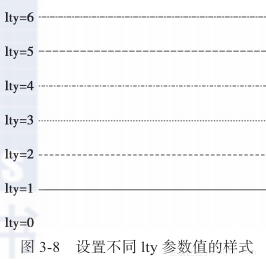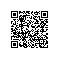# 《R语言游戏数据分析与挖掘》一3.1　常用图形参数

### 3.1　常用图形参数

R是一个功能强大的图形构建平台，可以逐条输入语句构建图形元素（颜色、点、线、文本等），逐渐完善图形，直至得到想要的结果。

#### 3.1.1　颜色元素

R语言可以设置绘图参数col，改变图像、坐标轴、文字、点、线等的颜色。例如，对数据集women绘制红色散点图，只需将col参数设置为"red"，如图3-1所示。

> plot(women,col="red") # 通过颜色名称设置散点颜色

> plot(women,col=554)        # 通过颜色下标设置
> plot(women,col="#FF0000")    #通过十六进制的颜色值设置
> mycolor<- rgb(red=255,green=0,blue=0,max=255)
> plot(women,col=mycolor)        # 通过RGB值设置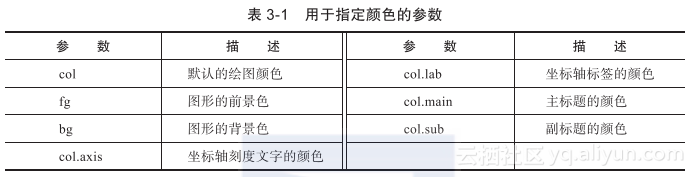> plot(women,main="身高 VS 体重散点图",sub="数据来源：women数据集",
+       col="red",col.main="green",col.sub="blue",
+       col.axis="grey",col.lab="yellow")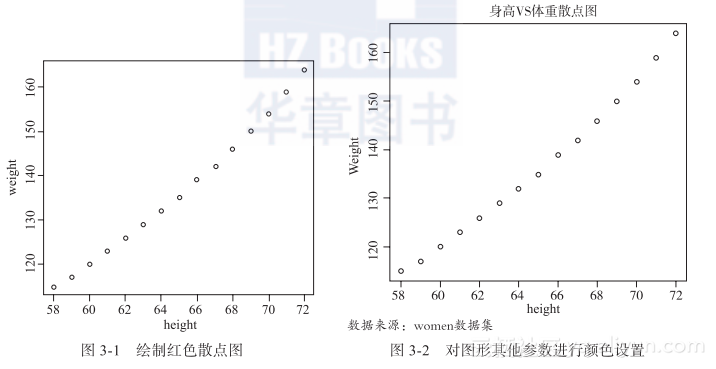R语言提供了自带的固定种类颜色，主要涉及的函数是colors()，该函数可以生成657种颜色名称，代表657种颜色，具体如下。

> colors()
 "white"                "aliceblue"            "antiquewhite"
 "antiquewhite1"        "antiquewhite2"        "antiquewhite3"
 "antiquewhite4"        "aquamarine"           "aquamarine1"
……
 "wheat3"             "wheat4"               "whitesmoke"
 "yellow"             "yellow1"              "yellow2"
 "yellow3"            "yellow4"              "yellowgreen"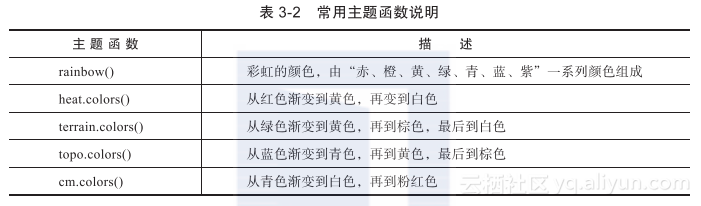> barplot(rep(1,7),col=rainbow(7),main="barplot(rep(1,7),col=rainbow(7))")
> barplot(rep(1,7),col=heat.colors(7),main="barplot(rep(1,7),col=heat.colors(7))")
> barplot(rep(1,7),col=terrain.colors(7),main="barplot(rep(1,7),col=terrain.colors(7))")
> barplot(rep(1,7),col=topo.colors(7),main="barplot(rep(1,7),col=topo.colors(7))")
> barplot(rep(1,7),col=cm.colors(7),main="barplot(rep(1,7),col=cm.colors(7))")
> par(mfrow=c(1,1))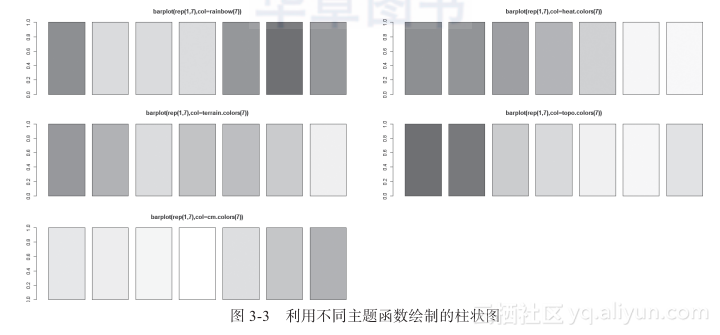#### 3.1.2　文字元素

> plot(0:4,type="n",axes = F,xlab = NA,ylab = NA)
> type <- c("正常字体(默认)","粗体字体","斜体字体","粗斜体字体")
> for(i in 1:4){
+   text(2,5-i,labels = paste0("font=",i,":",type[i]),font = i)
+ }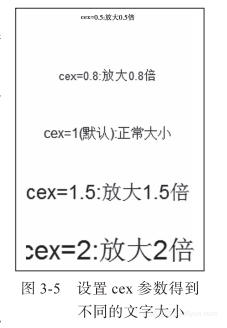> plot(0:5,type="n",axes = F,xlab = NA,ylab = NA)
> text(2,5,labels="cex=0.5:放大0.5倍",cex=0.5)
> text(2,4,labels="cex=0.8:放大0.8倍",cex=0.8)
> text(2,3,labels="cex=1(默认):正常大小",cex=1)
> text(2,2,labels="cex=1.5:放大1.5倍",cex=1.5)
> text(2,1,labels="cex=2:放大2倍",cex=2)


#### 3.1.3　点元素

pch（点样式）参数可取0～25的数字，对于符号21～25，还可以指定边界颜色(col=)和填充色(bg=)。下面代码中的pch取值为0～25，结果如图3-6所示。

> plot(1,col="white",xlim=c(1,7),ylim=c(1,5),
+        main = "点样式 pch=",xlab=NA,ylab=NA)
> for(i in c(0:25)){
+    x<-(i %/% 5)*1+1
+    y<-6-(i%%5)-1
+    if(length(which(c(21:25)==i)>=1)){
+     points(x,y,pch=i,col="blue",bg="yellow",cex=2)
+   } else {
+     points(x,y,pch=i,cex=2)
+   }
+   text(x+0.2,y,labels = i)
+ }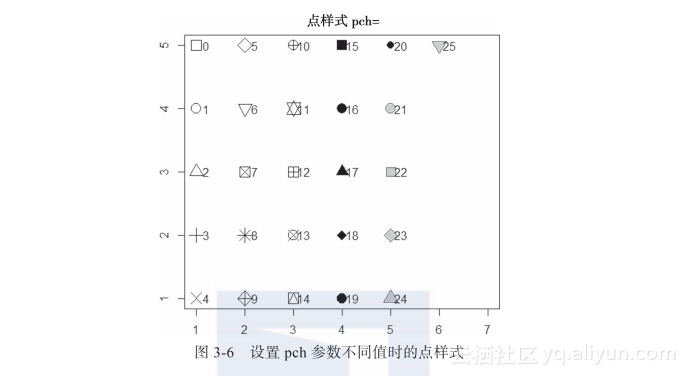> # pch取值可以为"*，？，a，A，0，.，+，-，|"
> points(6,4,pch="*",cex=2);text(6+0.2,4,labels="\"*\"")
> points(6,3,pch="?",cex=2);text(6+0.2,3,labels="\"?\"")
> points(6,2,pch="a",cex=2);text(6+0.2,2,labels="\"a\"")
> points(6,1,pch="A",cex=2);text(6+0.2,1,labels="\"A\"")
> points(7,5,pch="0",cex=2);text(7+0.2,5,labels="\"0\"")
> points(7,4,pch=".",cex=2);text(7+0.2,4,labels="\".\"")
> points(7,3,pch="+",cex=2);text(7+0.2,3,labels="\"+\"")
> points(7,2,pch="-",cex=2);text(7+0.2,2,labels="\"-\"")
> points(7,1,pch="|",cex=2);text(7+0.2,1,labels="\"|\"")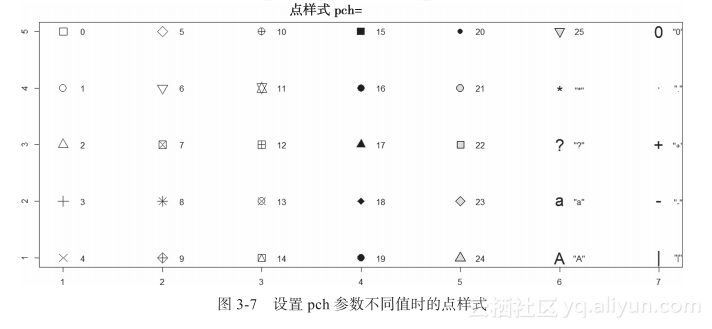#### 3.1.4　线元素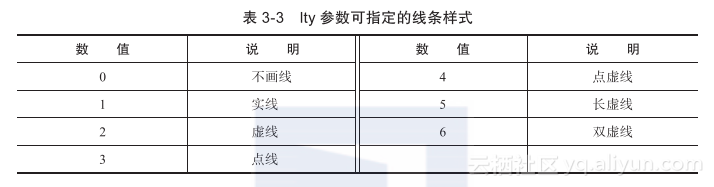> plot(x=1:10,y=rep(1,10),type="l",lty=0,ylim=c(1,8),xlim=c(-1,10),
+       axes=F,xlab=NA,ylab=NA)
> text(0,1,labels="lty=0")
> for(i in 2:7){
+   lines(x=1:10,y=rep(i,10),lty=i-1,xlab=NA, ylab=NA)
+   text(0,i,labels=paste0("lty=",i-1))
+ }